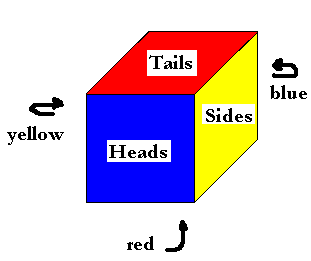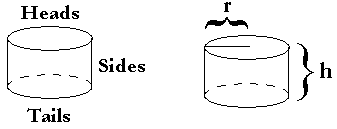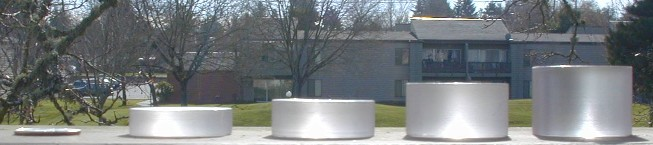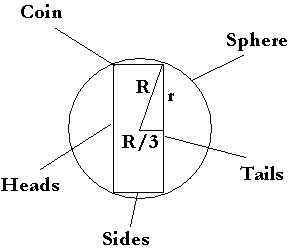# Constructing a fair 3 sided coin

3/21/05

For a 2 sided coin, the kind we are all familiar with, being "fair" means that P(Heads) = P(Tails) = .5. What would a 3 sided coin look like? Can a 3 sided coin be made "fair" so P(Heads) = P(Tails) = P(Sides) = 1/3? And how do we know fairness can even be obtained? This informal paper explores these questions.

A 3 sided "coin" can take several shapes. I put "coin" in quotes because it won't be a coin as we commonly use the term. Here are several possible ways a 3 sided coin look, with their outcomes, Heads, Tails, and Sides, labeled.

The first 3 sided coin I thought of was a long triangular prism. In the first version, each long face is an outcome, and the triangular edges could be rounded to prevent the coin from landing on the ends. In the second version, each triangular end is an outcome, and the long faces collectively count as one outcome.Some real life examples of this are the delicious Toblerone chocolate bars, and a ruler (which is not delicious).

Or, for example, these D3 dice

One could also make a 3 sided dreidel (please forgive my Paint skills!).Another example is a die with opposite sides painted the same colors to count as the same outcome.In other words, D6 dice with sides 1 through 3 twice are D3 dice. For example, these.

As interesting as these shapes are, I was more interested in maintaining the coin shape as much as possible, so I chose to focus on a right circular cylinder.

Here is a diagram of a right circular cylinder, our 3 sided coinDavid Boll made some postings on this topic on internet newsgroups, and I contacted him around 1998, saying that I'd be interested in flipping the coins he made. (Note, this link apparently is no longer working. See the last time David's page was updated on Archive.org here -Justin)

Here are the coins, or varying heights, that he made, with a quarter for referenceThe coins, going from left to right, not including the quarter: h = .5r, h = .75r, h = r, and h = 1.334r. David sent me the coins, and I ended up flipping three of these coins over 10,000 times total! (Note, this link apparently is no longer working. See the last time David's page on the 3 sided coins was updated on Archive.org here -Justin) From the data, it was determined that the coin where h = r was the most fair, with P(Sides) = 1240/3800 = .326. In David's own experiments, he obtained P(Sides) = 319/1000 = .319. Intuitively this makes sense, because having h = r makes the coin more die-like. However, the results could have been flukes.

It is important to note that it is not too clear how to fairly flip such a coin because we now have more than one axis of rotation to think about. When I flipped them, I tossed them, one at a time, as haphazardly as I could into a wall, and then the coin would fall on to a short carpet, sort of like rolling dice in a game of craps.

I set out to explore the mathematics behind these 3 sided coins. But first, what reason do we have in thinking fairness, that is, P(Heads) = P(Tails) = P(Sides) = 1/3, can be obtained?

For a 2 sided coin, h is small (see the quarter above), and therefore P(Sides) is very small. Conversely, think of a Pringles can, where h is very large, thus making P(Sides) very large. Therefore, it stands to reason that there is high probability that some h exists between the extremes where P(Sides) is "just right".

In Frederick Mosteller's Fifty Challenging Problems in Probability, Problem 38 reads (paraphrasing using my terminology of "height" for "thick" and "Sides" for "edge")

What height should a coin be to have a 1/3 chance of landing on Sides?

The story goes, the great mathematician John von Neumann solved this problem in his head and provided an answer to three decimal places, all in less than half a minute! Going by von Neumann's remarkable work, I tend to believe that story.

The solution: put the coin in a sphere, with the center of the coin being the center of the sphere, then select a random point on the surface of the sphere. Draw a straight line from this point to the center, and if that line hits the side of the coin, the coin can be considered to have landed on Sides.

Mosteller writes that a theorem from solid geometry simplifies this problem. Apparently when parallel planes cut a sphere, they produce an "orange-peel-like band" between the planes. This band is called a "zone". The surface area of a zone is proportional to the distance between the planes, and therefore our coin should have a height 1/3 of the sphere's height.Let R be the radius of the sphere and r the radius of the coin. The Pythagorean Theorem gives

R2 = r2+(1/9)R2

Solving for (2/3)R, which is h, gives

(2/3)R = (sqrt(2)/2)r

or h ~ .707r

Therefore, to obtain P(Heads) = P(Tails) = P(Sides) = 1/3, construct the coin so h = .707r. This theoretical result is surprising because it does not coincide with the experimental result of the best performing coin being where h = r. In fact, when I originally flipped the coins I obtained P(Sides) = 138/3800 = .046 using a coin where h = .75r, and David obtained P(Sides) = 88/800 = .11.

The discrepancies could be due to statistical flukes, the material of the coin, the material of where the coin was landing, the flipping method, the mathematical theory being too simple, or some combination of these.

For further detailed discussion of 3-sided coins, I strongly recommend the article Teaching Bayesian Model Comparison With the Three-Sided Coin by Scott Kuindersma and Brian Blais in The American Statistician, August 2007, Vol. 61, No. 3.

If you enjoyed any of my content, please consider supporting it in a variety of ways: